The OpenFOAM Foundation
Polynomial< PolySize > Class Template Reference

Polynomial templated on size (order): More...

Inheritance diagram for Polynomial< PolySize >: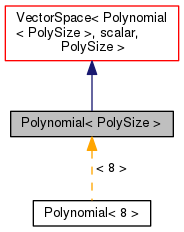[legend]
Collaboration diagram for Polynomial< PolySize >: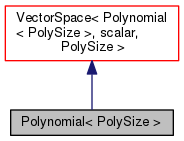[legend]

## Public Types

typedef Polynomial< PolySize > polyType

typedef Polynomial< PolySize+1 > intPolyTypePublic Types inherited from VectorSpace< Polynomial< PolySize >, scalar, PolySize >
typedef VectorSpace< Polynomial< PolySize >, scalar, Ncmpts > vsType
VectorSpace type. More...

typedef scalar cmptType
Component type. More...

## Public Member Functions

Polynomial ()
Construct null, with all coefficients = 0.0. More...

Polynomial (const Polynomial &)
Copy constructor. More...

Polynomial (const scalar coeffs[PolySize])
Construct from C-array of coefficients. More...

Polynomial (const UList< scalar > &coeffs)
Construct from a list of coefficients. More...

Polynomial (Istream &)
Construct from Istream. More...

Polynomial (const word &name, Istream &)
Construct from name and Istream. More...

bool logActive () const
Return true if the log term is active. More...

scalar logCoeff () const
Return the log coefficient. More...

scalar value (const scalar x) const
Return polynomial value. More...

scalar derivative (const scalar x) const
Return derivative of the polynomial at the given x. More...

scalar integral (const scalar x1, const scalar x2) const
Return integral between two values. More...

intPolyType integral (const scalar intConstant=0.0) const
Return integral coefficients. More...

polyType integralMinus1 (const scalar intConstant=0.0) const
Return integral coefficients when lowest order is -1. More...Public Member Functions inherited from VectorSpace< Polynomial< PolySize >, scalar, PolySize >
VectorSpace ()
Construct null. More...

VectorSpace (const Foam::zero)
Construct initialized to zero. More...

VectorSpace (Istream &)
Construct from Istream. More...

VectorSpace (const VectorSpace< Polynomial< PolySize >, scalar, Ncmpts > &)
Construct as copy. More...

VectorSpace (const VectorSpace< Form2, Cmpt2, Ncmpts > &)
Construct as copy of a VectorSpace with the same size. More...

const scalar & component (const direction) const

scalar & component (const direction)

void component (scalar &, const direction) const

void replace (const direction, const scalar &)

const ConstBlock< SubVector, BStart > block () const

const scalar & operator[] (const direction) const

scalar & operator[] (const direction)

void operator= (const VectorSpace< Polynomial< PolySize >, scalar, Ncmpts > &)

void operator= (const Foam::zero)

void operator+= (const VectorSpace< Polynomial< PolySize >, scalar, Ncmpts > &)

void operator-= (const VectorSpace< Polynomial< PolySize >, scalar, Ncmpts > &)

void operator*= (const scalar)

void operator/= (const scalar)

## Friends

Ostreamoperator (Ostream &, const Polynomial &)
Ostream Operator. More...Static Public Member Functions inherited from VectorSpace< Polynomial< PolySize >, scalar, PolySize >
static direction size ()
Return the number of elements in the VectorSpace = Ncmpts. More...

static Polynomial< PolySize > uniform (const scalar &s)
Return a VectorSpace with all elements = s. More...Public Attributes inherited from VectorSpace< Polynomial< PolySize >, scalar, PolySize >
scalar v_ [Ncmpts]
The components of this vector space. More...Static Public Attributes inherited from VectorSpace< Polynomial< PolySize >, scalar, PolySize >
static const direction dim
Dimensionality of space. More...

static const direction nComponents
Number of components in this vector space. More...

static const direction mRows

static const direction nCols

static const char *const typeName

static const char *const componentNames []

static const Polynomial< PolySize > zero

static const Polynomial< PolySize > one

static const Polynomial< PolySize > max

static const Polynomial< PolySize > min

static const Polynomial< PolySize > rootMax

static const Polynomial< PolySize > rootMin

## Detailed Description

### template<int PolySize> class Foam::Polynomial< PolySize >

Polynomial templated on size (order):

poly = sum(coeff_[i]*x^i) logCoeff*log(x)

where 0 <= i <= N

• integer powers, starting at zero
• value(x) to evaluate the poly for a given value
• derivative(x) returns derivative at value
• integral(x1, x2) returns integral between two scalar values
• integral() to return a new, integral coeff polynomial
• increases the size (order)
• integralMinus1() to return a new, integral coeff polynomial where the base poly starts at order -1
Source files

Definition at line 63 of file Polynomial.H.

## Member Typedef Documentation

 typedef Polynomial polyType

Definition at line 96 of file Polynomial.H.

 typedef Polynomial intPolyType

Definition at line 98 of file Polynomial.H.

## Constructor & Destructor Documentation

 Polynomial ( )

Construct null, with all coefficients = 0.0.

Definition at line 31 of file Polynomial.C.

 Polynomial ( const Polynomial< PolySize > & )

Copy constructor.

 Polynomial ( const scalar coeffs[PolySize] )
explicit

Construct from C-array of coefficients.

Definition at line 57 of file Polynomial.C.

 Polynomial ( const UList< scalar > & coeffs )
explicit

Construct from a list of coefficients.

Definition at line 71 of file Polynomial.C.

Here is the call graph for this function: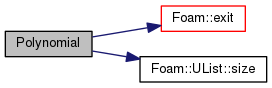Polynomial ( Istream & is )

Construct from Istream.

Definition at line 93 of file Polynomial.C.

 Polynomial ( const word & name, Istream & is )

Construct from name and Istream.

Definition at line 102 of file Polynomial.C.

Here is the call graph for this function: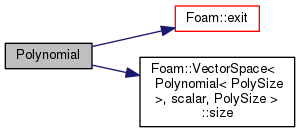## Member Function Documentation

 bool logActive ( ) const

Return true if the log term is active.

Definition at line 132 of file Polynomial.C.

 Foam::scalar logCoeff ( ) const

Return the log coefficient.

Definition at line 139 of file Polynomial.C.

 Foam::scalar value ( const scalar x ) const

Return polynomial value.

Definition at line 146 of file Polynomial.C.

Here is the call graph for this function: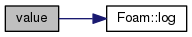Foam::scalar derivative ( const scalar x ) const

Return derivative of the polynomial at the given x.

Definition at line 168 of file Polynomial.C.

Here is the call graph for this function: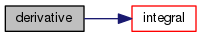Foam::scalar integral ( const scalar x1, const scalar x2 ) const

Return integral between two values.

Definition at line 196 of file Polynomial.C.

Referenced by Polynomial< PolySize >::derivative().

Here is the call graph for this function: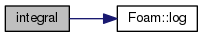Here is the caller graph for this function: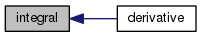Foam::Polynomial< PolySize >::intPolyType integral ( const scalar intConstant = `0.0` ) const

Return integral coefficients.

Argument becomes zero'th element (constant of integration)

Definition at line 224 of file Polynomial.C.

 Foam::Polynomial< PolySize >::polyType integralMinus1 ( const scalar intConstant = `0.0` ) const

Return integral coefficients when lowest order is -1.

Argument becomes zero'th element (constant of integration)

Definition at line 240 of file Polynomial.C.

## Friends And Related Function Documentation

 Ostream& operator ( Ostream & , const Polynomial< PolySize > & )
friend

The documentation for this class was generated from the following files: